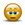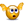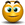# Regression Analysis for memory retention data

## Excel Help for Regression Analysis For Memory Retention Data in Analyzing Corporate Financial Data

### Regression Analysis For Memory Retention Data

Rate this:
(3/5 from 1 vote)I'm super hopeless at maths and need some help. Find the average percentage memory retention (p) measured against passing time (t, in minutes).I need to construct:A scatter plot of p against log(t). The graph needs to indicate a linear straight line relation between average percentage memory retention (p) and passign time (t, in minutes)Add a trend line to add a linear regression line of the form of y=mx+b, where m is the slope and b is the y-interceptCalculate the regression of p using given x values of t. for example p-reg=m*log(t)+bData set below:t minutes            Avg % mem retention (p)1                        0.845                        0.7115                      0.6130                      0.5660                      0.54120                    0.47240                    0.45480                    0.38720                    0.361440                  0.262880                  0.205760                  0.1610080                0.08Does this make sense to anyone??? Please help...thanx Posted by CITHelp on 25 Sep 2010
 Replies - Displaying 1 to 5 of 5 Order Replies By: Most Recent | Chronological | Highest RatedRate this: (3/5 from 1 vote) In this case, you can start by creating a simple XY scatter plot chart from the source data. By selecting the series in the chart you can right click and select Add Trend line > Linear.  Right click the Trend Line and choose Form Trend line > Options and choose to add the equation and R-squared on the chart.  This will give you the regression equation and the predictive quality (R-squared) for your analysis.To transform or analyze the data before charting, you can use the LOG function and/or other statistical functions such as SLOPE (regression coefficient) under the Statistical functions in Excel. Excel Business Forums Administrator Posted by Excel Helper on 25 Sep 2010Rate this: (3/5 from 1 vote) Hi,Thanx for that...You rock!. I have created the scatter plot graph. However, i'm still stuck!How do i find log(t) and rep-p??? It's probably very obvious but i can't seem to get the formula.thanxCITHelp Posted by CITHelp on 26 Sep 2010Rate this: (3/5 from 1 vote) Instead of adding the linear trend line to the scatter plot series, you can add a logarithmic trend line.  When displaying the equation and r-squared, you will get the Log(t) (where "x" replaces t) and the p-reg is represented by "y" in the equation as the dependent variable of the regression equation. Excel Business Forums Administrator Posted by Excel Helper on 26 Sep 2010Rate this: (3/5 from 1 vote) Hi Excel Helper,Thanx so much for your help. I need to pick your brain again.For each of the data lines i need to find log(t) and reg-p....how do i do this???t minutes            Avg % mem retention (p)        log(t)    reg-p1                        0.84                                      ?           ?5                        0.71                                      ?           ?15                      0.61                                      ?           ?30                      0.56                                      ?           ?60                      0.54                                      ?           ?120                    0.47                                      ?           ? etc240                    0.45480                    0.38720                    0.361440                  0.262880                  0.205760                  0.1610080                0.08 Posted by CITHelp on 27 Sep 2010Rate this: (3/5 from 1 vote) The view plot the predicted log(t) and p, simply use the calculated regression equation with the original input values. Excel Business Forums Administrator Posted by Excel Helper on 27 Sep 2010
 Displaying page 1 of 1

 Find relevant Excel templates and add-ins for Regression Analysis for memory retention data in the Excel Business Solutions Directory